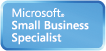Welcome Guest, you are in: Login

MUST Creative Engineering Laboratory

Technical Doc

Search the wiki
»

www.must.or.krNeural Networks

Modified on 2010/05/17 11:51 Categorized as Data Mining

Neural Networks

• Analogy to Biological Systems (Indeed a great example of a good learning system)
• Massive Parallelism allowing for computational efficiency
• The first learning algorithm came in 1959 (Rosenblatt) who suggested that if a target output value is provided for a single neuron with fixed inputs, one can incrementally change weights to learn to produce these outputs using the perceptron learning rule

A Neuron• The n-dimensional input vector x is mapped into variable y by means of the scalar product and a nonlinear function mappingFor ExampleMulti-Layer PerceptronNetwork Training

• The ultimate objective of training
obtain a set of weights that makes almost all the tuples in the training data classified correctly

Steps Initialize weights with random values Feed the input tuples into the network one by one For each unit Compute the net input to the unit as a linear combination of all the inputs to the unit Compute the output value using the activation function Compute the error Update the weights and the bias

Network Pruning and Rule Extraction

Network pruning Fully connected network will be hard to articulate N input nodes, h hidden nodes and m output nodes lead to h(m+N) weights Pruning: Remove some of the links without affecting classification accuracy of the network Extracting rules from a trained network Discretize activation values; replace individual activation value by the cluster average maintaining the network accuracy Enumerate the output from the discretized activation values to find rules between activation value and output Find the relationship between the input and activation value Combine the above two to have rules relating the output to input